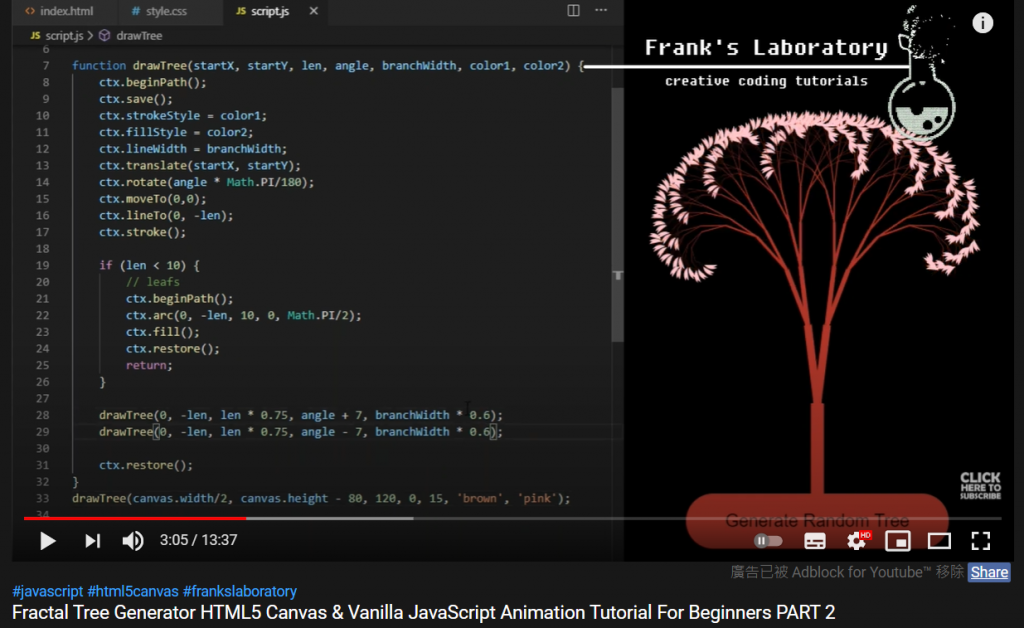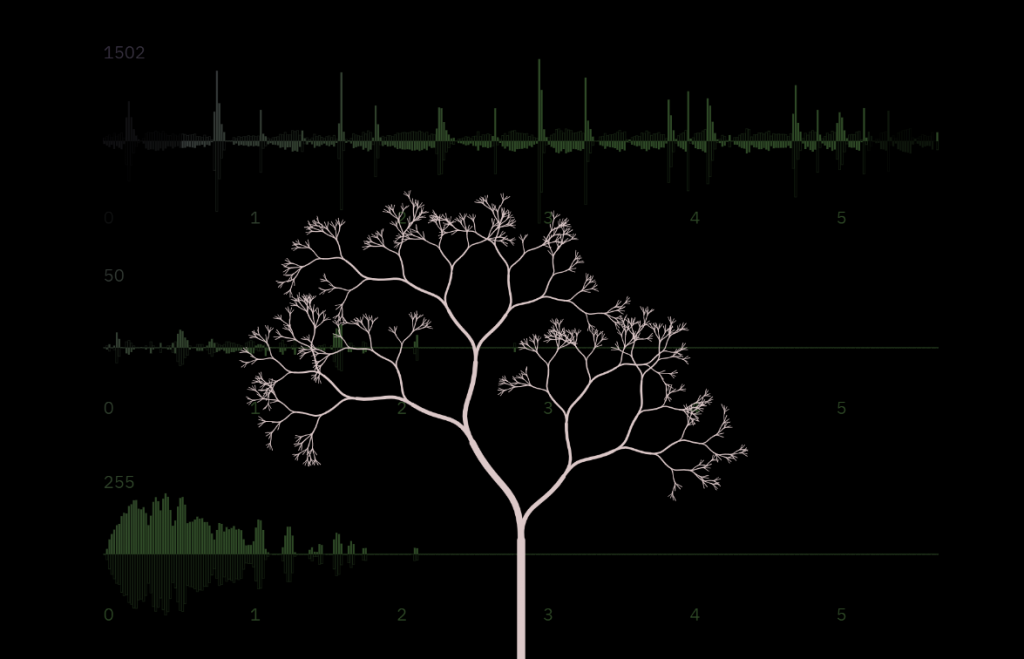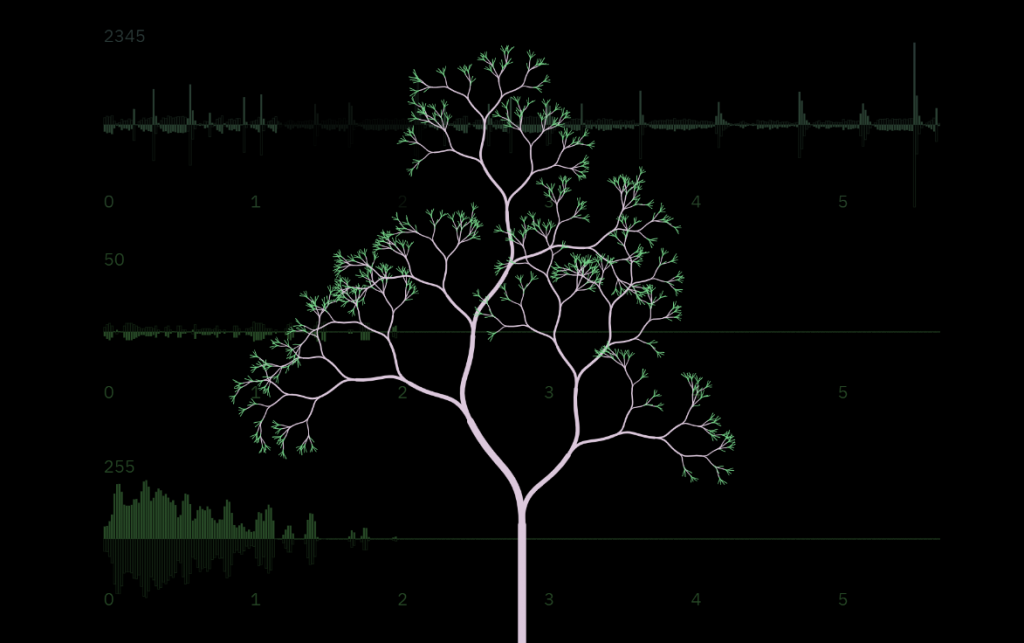#DAY 26
0
Modern Web

## 讓我們說回那顆樹

Fractal Tree Part1
Fractal Tree Part2## 修飾樹的形狀``````for(let N = 1; N < treeNodes.length; N++){
let node = treeNodes[N];
let theta1 = node.father.theta / 180 * Math.PI,
theta2 = node.theta / 180 * Math.PI;
}
``````

``````x1 = x0 + 0.5 * r * Math.cos(theta1)
y1 = y0 - 0.5 * r * Math.sin(theta1)
``````

``````x2 = x0 + 0.5 * r * Math.cos(theta2)
y2 = y0 - 0.5 * r * Math.sin(theta2)
``````

``````x3 = x0 + r * Math.cos(theta2)
y3 = y0 - r * Math.sin(theta2)
``````

``````Tree.prototype.Draw = function(){
for(let N = 1; N < treeNodes.length; N++){
let node = treeNodes[N];
let x = node.father.endX,
y = node.father.endY,
r = node.r * Math.pow(node.grow, 1 + 3 * N/treeNodes.length),
theta1 = node.father.theta / 180 * Math.PI,
theta2 = node.theta / 180 * Math.PI;
context.beginPath();
context.moveTo(x, y);
context.bezierCurveTo(x + 0.5 * r * Math.cos(theta1),
y - 0.5 * r * Math.sin(theta1),
x + 0.5 * r * Math.cos(theta2),
y - 0.5 * r * Math.sin(theta2),
x + r * Math.cos(theta2),
y - r * Math.sin(theta2));
context.lineWidth = 0.5 + Math.pow(r, 1.1)/30;
context.strokeStyle = 'rgba(220, 200, 200, 1)';
context.stroke();
}
}
``````

`r = node.r * Math.pow(node.grow, 1 + 3 * N/treeNodes.length)`是讓樹枝依序(遞迴的順序)長出來的一個新寫法，還沒下定論，所以不特別討論

## 樹的分支

``````let Stick = function(father, shrink_diff, angleOffset, times){
this.father = father;
this.r = this.father.r * (shrink_diff);
this.theta = this.father.theta + angleOffset;
if(this.r < 20 || times < 0){
return this;
}
// 以下略
// ......
}
``````

``````let shrink = 0.65 + random(0.1);
let diff = random(0.3) - 0.15; // +-0.15
if(this.r > 40)
this.son = [new Stick(this, (shrink - diff), 30 * (diff + 1), times - 1),
new Stick(this, (shrink + diff), 30 * (diff - 1), times - 1)];
else
this.son = [
new Stick(this, (shrink - diff), 30 * (diff + 1), times - 1),
new Stick(this, (shrink + diff), 30 * (diff - 1), times - 1),
new Stick(this, (0.7 + random(0.1)), 30 * ( 0.5 + diff), times - 1),
new Stick(this, (0.7 - random(0.1)), 30 * (-0.5 - diff), times - 1)];
``````